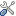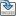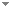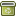# The $L\Pi$ and $L\Pi\frac{1}{2}$ logics: two complete fuzzy systems joining Łukasiewicz and Product Logics [Book Review]

Archive for Mathematical Logic 40 (1):39-67 (2001)

 Abstract In this paper we provide a finite axiomatization (using two finitary rules only) for the propositional logic (called $L\Pi$ ) resulting from the combination of Lukasiewicz and Product Logics, together with the logic obtained by from $L \Pi$ by the adding of a constant symbol and of a defining axiom for $\frac{1}{2}$ , called $L \Pi\frac{1}{2}$ . We show that $L \Pi \frac{1}{2}$ contains all the most important propositional fuzzy logics: Lukasiewicz Logic, Product Logic, Gödel's Fuzzy Logic, Takeuti and Titani's Propositional Logic, Pavelka's Rational Logic, Pavelka's Rational Product Logic, the Lukasiewicz Logic with $\Delta$ , and the Product and Gödel's Logics with $\Delta$ and involution. Standard completeness results are proved by means of investigating the algebras corresponding to $L \Pi$ and $L \Pi \frac{1}{2}$ . For these algebras, we prove a theorem of subdirect representation and we show that linearly ordered algebras can be represented as algebras on the unit interval of either a linearly ordered field, or of the ordered ring of integers, Z Keywords Legacy Categories (categorize this paper) DOI 10.1007/s001530050173 OptionsEdit this recordMark as duplicateExport citationFind it on ScholarRequest removal from indexRevision history

PhilArchive copy

Upload a copy of this paper     Check publisher's policy     Papers currently archived: 71,231

Setup an account with your affiliations in order to access resources via your University's proxy server
Configure custom proxy (use this if your affiliation does not provide a proxy)

## References found in this work BETA

No references found.

## Citations of this work BETA

On Theories and Models in Fuzzy Predicate Logics.Petr Hájek & Petr Cintula - 2006 - Journal of Symbolic Logic 71 (3):863 - 880.
On Vagueness, Truth Values and Fuzzy Logics.Petr Hájek - 2009 - Studia Logica 91 (3):367-382.
Mathematical Fuzzy Logics.Siegfried Gottwald - 2008 - Bulletin of Symbolic Logic 14 (2):210-239.

## Similar books and articles

Advances in the ŁΠ and Logics.Petr Cintula - 2003 - Archive for Mathematical Logic 42 (5):449-468.
An Algebraic Approach to Propositional Fuzzy Logic.Franco Montagna - 2000 - Journal of Logic, Language and Information 9 (1):91-124.
Commutative Basic Algebras and Non-Associative Fuzzy Logics.Michal Botur & Radomír Halaš - 2009 - Archive for Mathematical Logic 48 (3-4):243-255.
Structural Completeness in Fuzzy Logics.Petr Cintula & George Metcalfe - 2009 - Notre Dame Journal of Formal Logic 50 (2):153-182.
Substructural Fuzzy Logics.George Metcalfe & Franco Montagna - 2007 - Journal of Symbolic Logic 72 (3):834 - 864.
Product Ł Ukasiewicz Logic.Rostislav Horčík & Petr Cintula - 2004 - Archive for Mathematical Logic 43 (4):477-503.
Pavelka-Style Completeness in Expansions of Łukasiewicz Logic.Hector Freytes - 2008 - Archive for Mathematical Logic 47 (1):15-23.
On Theories and Models in Fuzzy Predicate Logics.Petr Hájek & Petr Cintula - 2006 - Journal of Symbolic Logic 71 (3):863 - 880.
Strict Core Fuzzy Logics and Quasi-Witnessed Models.Marco Cerami & Francesc Esteva - 2011 - Archive for Mathematical Logic 50 (5-6):625-641.
Mathematical Fuzzy Logics.Siegfried Gottwald - 2008 - Bulletin of Symbolic Logic 14 (2):210-239.